# Team:SCU-China/modeling/algorithm

Team:SCU-China - 2018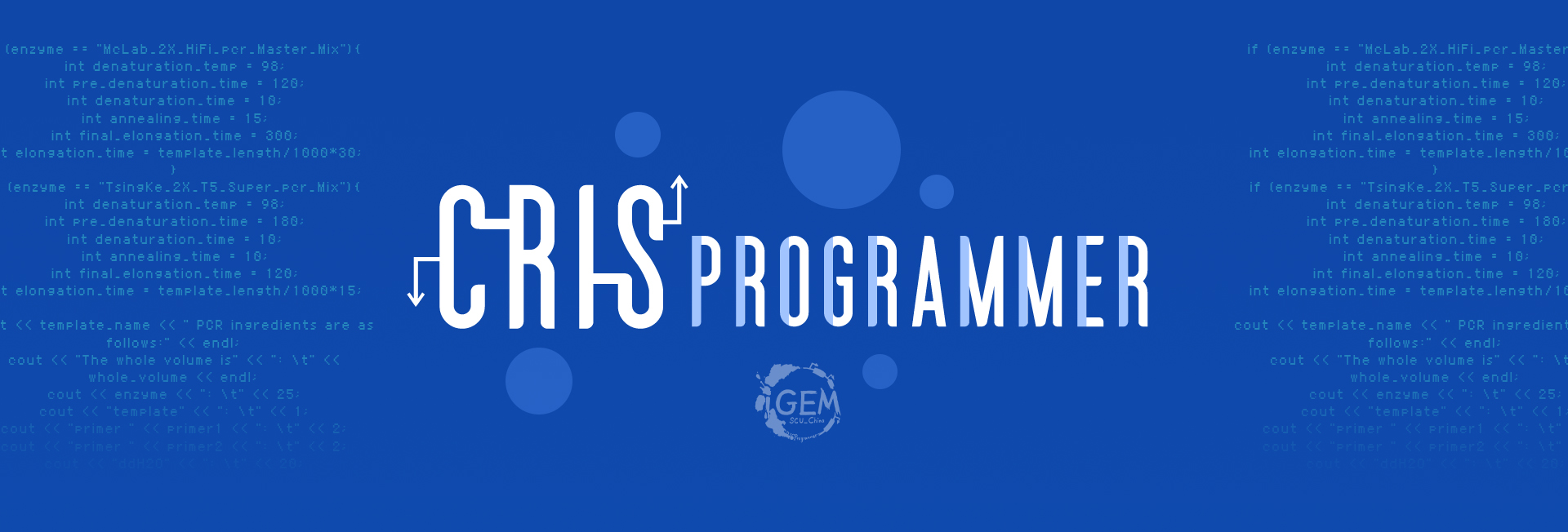In our experiment, we need to construct a sgRNA sequence that is orthogonal to both the genome and the plasmid. The algorithm steps are as follows:
1. Sequence summary: E.coli genome is sequence α, with length m; All plasmid sequences that need to be orthogonal are spliced into a sequence β of length n.
2. Genome orthogonal screening: A randomly generated sgRNA sequence λ that ends in NGG together with all 23 consecutive sequences on sequence α are ruled as heterotopic or, and their total weighted scores are obtained. Then reverse α and do the same thing. Eventually, we can get two score sequences of length m-22. The distribution function with the total weight points in the entire sample space was calculated in advance by means of parameter estimation, and then different threshold values of the orthogonal exponential threshold μ_1were selected according to different orthogonality requirements. The threshold value used in this experiment was 63 (90%). Retrieve two socre sequences.If there is a score value below μ1, it is considered not to satisfy genomic orthogonality;if the conditions are met, proceed to the next step. In addition, we used some empirical weight values - Weights 1 to 10 are assigned at a time from 10 digits away from the PAM end,10 in the seed region;t he 10 sites in the seed region assign an average value of 10; The 21st bit weight is 0,22 and 23 bit weight is 20.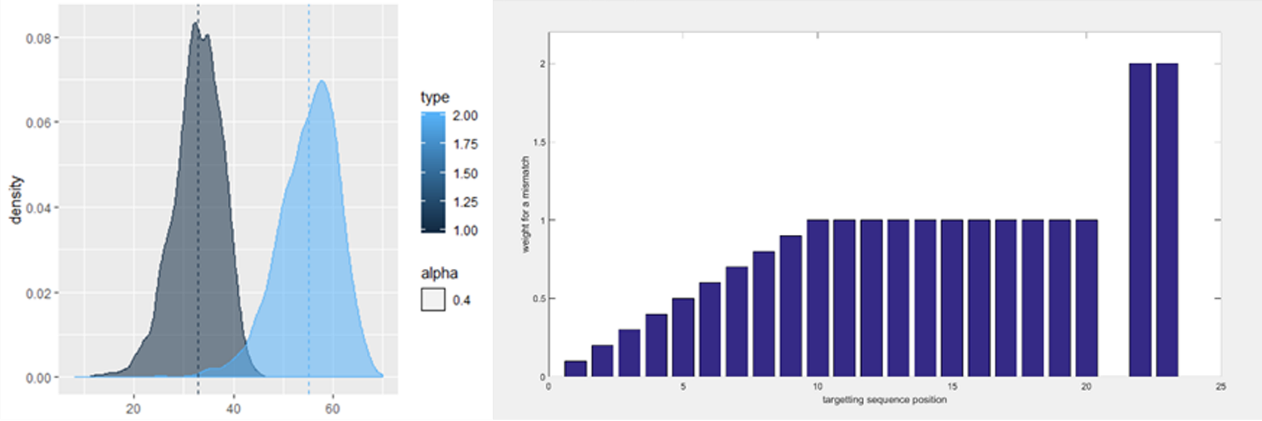Fig i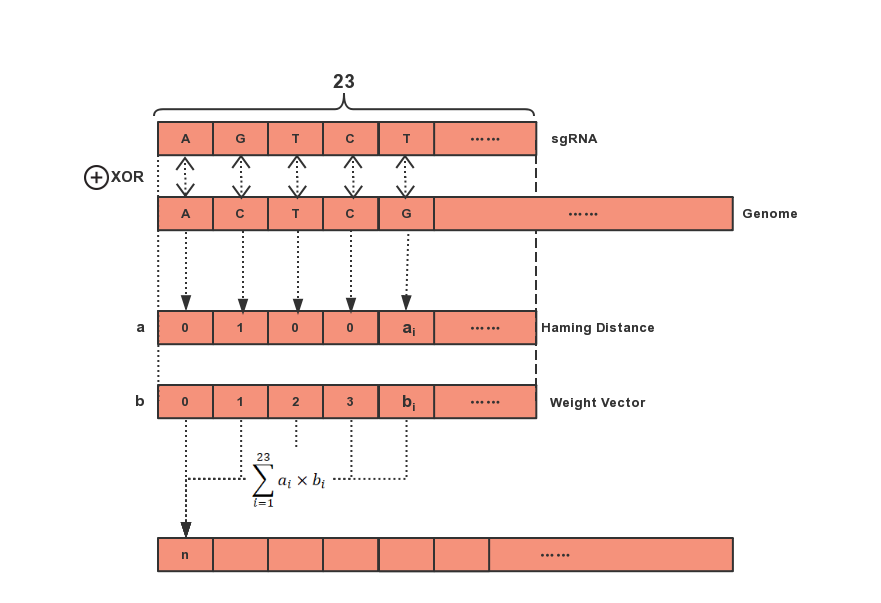Fig 2
3. Plasmid orthogonal screening: Repeat algorithm in step 2 using the sequence from step 2 and sequence β. And remove the weight score of two plasmid spliced sequences that do not exist in the bacteria.The screening was done with threshold value μ_2 = 43.Record the filtered sequence.
4. Repeat steps 1, 2, and 3 until the number of recorded sequences reaches 600.
5. Verify the orthogonality of all the obtained sequences among each other, and finally obtain the 20 sequences that are most orthogonal to the genome and plasmid. The result matrix obtained by mutual verification is as follows: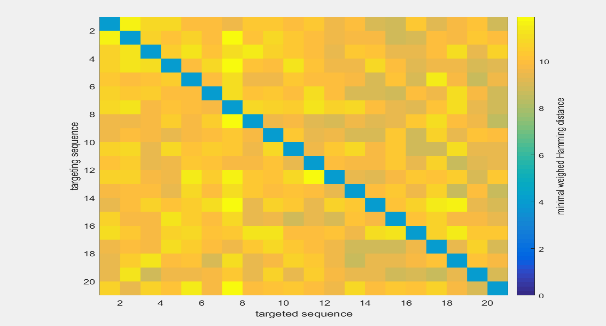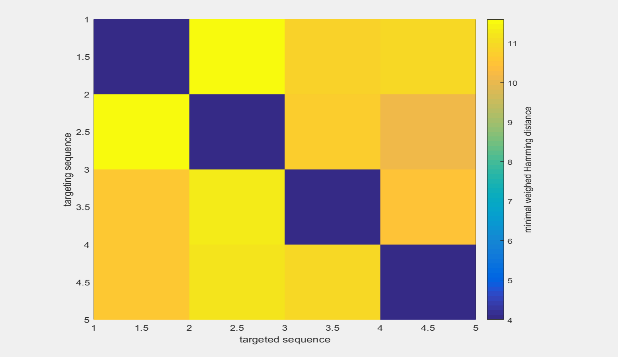Fig 3
Each pane shows the value of the orthogonality between two sequences.So it is clear we should choose the sequences on the top left.
All codes were written in Fortran and can be find here.

References

 Bikard, D., Jiang, W., Samai, P., Hochschild, A., Zhang, F., & Marraffini, L. A. (2013). Programmable repression and activation of bacterial gene expression using an engineered crispr-cas system. Nucleic Acids Research, 41(15), 7429-7437.